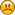###Author Topic: solving equations  (Read 3094 times)

#### venkat0249

• Newbie
•• Posts: 22##### solving equations
« on: September 16, 2011, 05:13:15 AM »
Is it possible to solve equations using hypernext? If not can we solve using Rb script?
For example: x+y=10, x+2y=13 then find x and y values.

#### tigabyte

• Newbie
•• Posts: 54##### Re: solving equations
« Reply #1 on: September 16, 2011, 05:59:04 AM »
Is it possible to solve equations using hypernext? If not can we solve using Rb script?
For example: x+y=10, x+2y=13 then find x and y values.

Yes you can use either but of course RBscript is much faster that HyperNext but HyperNext script is much easier to use

Here is a link with an algorithm:-

#### venkat0249

• Newbie
•• Posts: 22##### Re: solving equations
« Reply #2 on: September 16, 2011, 01:03:22 PM »
Thank you for the answer. Unfortunately the link is not workingcan you give me any other link?

#### tigabyte

• Newbie
•• Posts: 54##### Re: solving equations
« Reply #3 on: September 16, 2011, 01:36:48 PM »
Thank you for the answer. Unfortunately the link is not workingcan you give me any other link?

Their site is very slow to respond.

Here is the HyperNext based on their text - it gives the same answer for their problem.
Sorry I don't have time to tidy it up etc but their page should clarify it.
The script goes into a button and the answers for x and y appear in fields 1 and 2 respectively.

Code: [Select]
`Local a,b,c,d,e,f,x,yLocal t1,t2,t3,t4@'ax+by=c equation format will be like this@'dx+ey=f@x = (c * e - b * f) / (a * e - b * d)@y = (a * f - c * d) / (a * e - b * d)Put 1 into aPut 1 into bPut 3 into cPut 2 into dPut 3 into ePut 8 into f@ calc c@x = (c * e - b * f) / (a * e - b * d)Put c into  t1Multiply t1 by ePut b into t2Multiply t2 by fSubtract t2 from t1Put a into t3Multiply t3 by ePut b into t4Multiply t4 by dSubtract t4 from t3Put t1 into xDivide x by t3@ calc y@y = (a * f - c * d) / (a * e - b * d)Put a into t1Multiply t1 by fPut c into t2Multiply t2 by dSubtract t2 from t1Put a into t3Multiply t3 by ePut b into t4Multiply t4 by dSubtract t4 from t3Put t1 into yDivide y by t3Put x into field 1Put y into field 2`

#### venkat0249

• Newbie
•• Posts: 22##### Re: solving equations
« Reply #4 on: September 16, 2011, 03:47:40 PM »
Thank you soooo much....anything